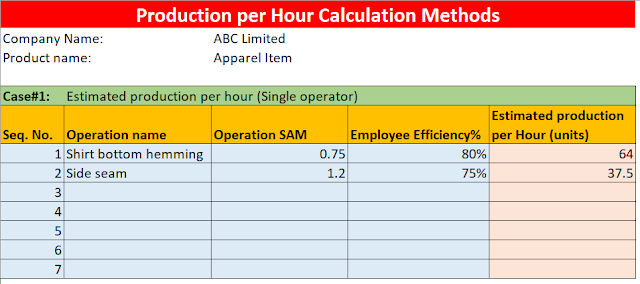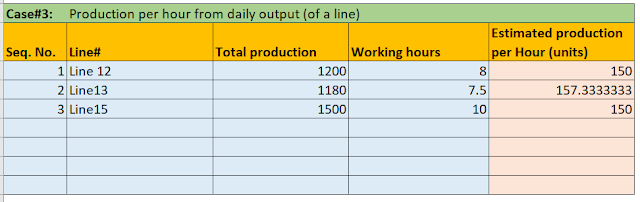# How to calculate production per hour in Excel?

Calculation of hourly production is required for many purposes. In the manufacturing sectors whatever items you are making, often you need to estimated hourly production and sometimes you may need to convert total production volume done by an employee (or workstation) into production per hour.

In the post, I will be showing you how you can calculate the estimated production per hour of a line in an Excel sheet. Then, I will show you the estimated production from each workstation (each operator). I will use examples of the garment manufacturing sector. I will be sharing an Excel template for calculating production per hour. At the end of this post, I have given Excel file download link.

Estimated production per hour from a workstation is calculated based on operators’ skills and operation cycle time. OR if you know the standard time of a specific operation and you know the skill level of the operator, we can estimate production without doing a cycle time study.

Related post: How to calculate the hourly production target of a production line?

### 1. Calculate estimated production per hour at target efficiency%.

In the following example, I have shown it at 100% and Target efficiency (60%). Formula used -

Calculated Production/Hour @ target efficiency = (60/Operation standard time) X Target Efficiency%

When you use this method, you need the following information
• standard time of the operation,
• expected performance rate (efficiency percentage).
Let’s say Satish is working on an operation of a standard time of 0.75 minutes. His performance rate (efficiency) is 80%.

Production per hour = (60/0.75) x 80%= 64 pieces of garmentsImages: Production per hour calculation template

### 2. Calculate estimated production per hour (with multiple workstations)

It is calculated according to the machine assigned to each operation. It may be different for few operations than calculated production per hour as you set machine numbers to round up value. Formula used -

Estimated Production/Hour = (No. of machine assigned * Hourly target qty)

In case, you have assigned more than one machine for a single operation, and you know the operator’s average efficiency, then you can estimate total production in that specific operation.

Example: Let’s say on the shirt bottom operation, you have assigned 3 machines and 3 operators. So hourly production rate from this operation will be 3 times the single operator’s output.

Production per hour = 64 x 3 = 192 pieces of garments.### 3. Production per hour from the total production quantity

Finally, I will show you how to know production per hour from the total production quantity and number of hours worked.

Let’s say a line produced 1200 garments in a day and line worked 8 hours.

So, here production per hour quantity of a production line will be equal to 1200/8 = 150 units of garments.

This production quantity can be operation-specific or of line output (assembled item output). You need to be specific when mentioning the production by hour quantity.

In another example, if you know total production of individual workers and have captured their total working hours in a day.   Calculate their hourly production rate using the following sheet.### Free Excel template

The free Excel template for calculating production per hour of a factory, line, and employee is here.